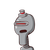# de = 14Find the area of a square park whose perimeter is 72m​

de = 14
Find the area of a square park whose perimeter is 72m​

### 1 thought on “de = 14<br />Find the area of a square park whose perimeter is 72m​”

1.Step-by-step explanation:

Perimeter of a square= 4a

72m= 4a

a= 72m/4= 18m

area= s²= 18m×18m

= 324m²